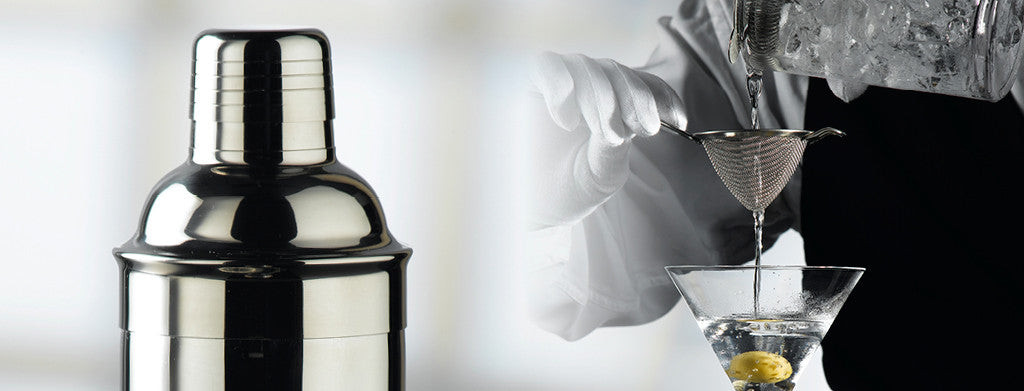Shopping Cart

### Refine

View all

#### Price# Artbar£3.58 each (ex VAT)£6.82 each (ex VAT)£11.93 each (ex VAT)£15.04 each (ex VAT)£6.58 each (ex VAT)£9.36 each (ex VAT)£31.99 each (ex VAT)£31.99 each (ex VAT)£32.93 each (ex VAT)£33.96 each (ex VAT)£8.96 each (ex VAT)£0.61 each (ex VAT)£4.53 each (ex VAT)£16.23 each (ex VAT)£13.49 each (ex VAT)£9.12 each (ex VAT)£9.95 each (ex VAT)£18.70 each (ex VAT)£11.71 each (ex VAT)£16.88 each (ex VAT)£2.93 each (ex VAT)£23.02 each (ex VAT)£13.85 each (ex VAT)£8.99 each (ex VAT)£2.43 each (ex VAT)£5.84 each (ex VAT)£4.14 each (ex VAT)£13.21 each (ex VAT)£5.96 each (ex VAT)£22.01 each (ex VAT)£7.22 each (ex VAT)£3.64 each (ex VAT)